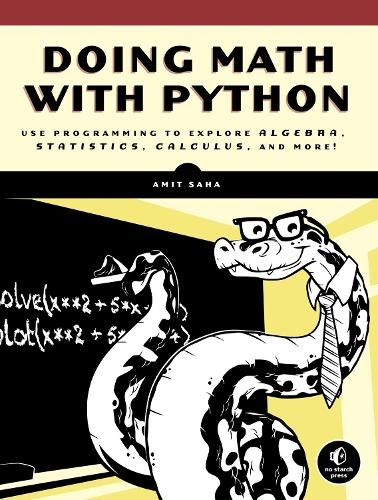•# Doing Math With Python (Paperback)

(author)
£24.99
Paperback 264 Pages / Published: 08/01/2015
• We can order this from the publisher

Usually dispatched within 10 working days

Doing Math with Python shows you how to use Python to delve into high school level math topics like statistics, geometry, probability, and calculus. You ll start with simple projects, like a factoring program and a quadratic-equation solver, and then create more complex projects once you ve gotten the hang of things. Along the way, you ll discover new ways to explore math and gain valuable programming skills that you ll use throughout your study of math and computer science. Learn how to: Describe your data with statistics, and visualize it with line graphs, bar charts, and scatter plots Explore set theory and probability with programs for coin flips, dicing, and other games of chance Solve algebra problems using Python s symbolic math functions Draw geometric shapes and explore fractals like the Barnsley fern, the Sierpinski triangle, and the Mandelbrot set Write programs to find derivatives and integrate functions Creative coding challenges and applied examples help you see how

Publisher: No Starch Press,US
ISBN: 9781593276409
Number of pages: 264
Weight: 518 g
Dimensions: 234 x 178 x 17 mm

MEDIA REVIEWS
"Saha does an excellent job providing a clear link between Python and upper-level math concepts, and demonstrates how Python can be transformed into a mathematical stage. This book deserves a spot on every geometry teacher's bookshelf."
-School Library Journal